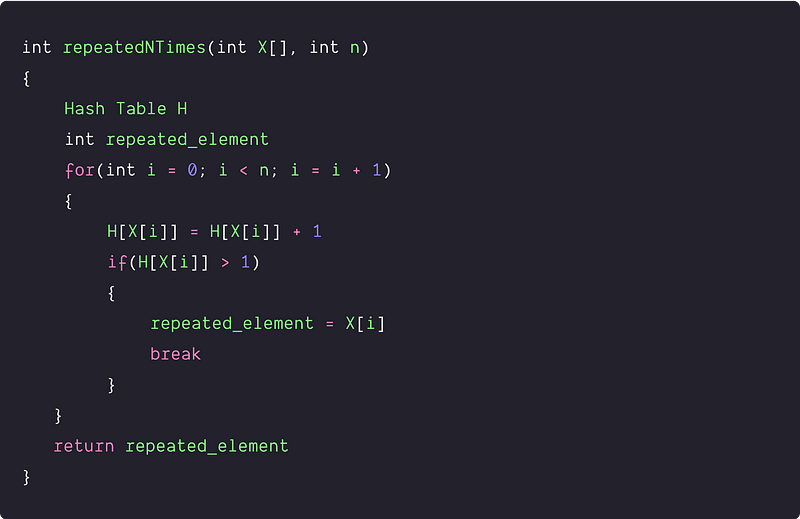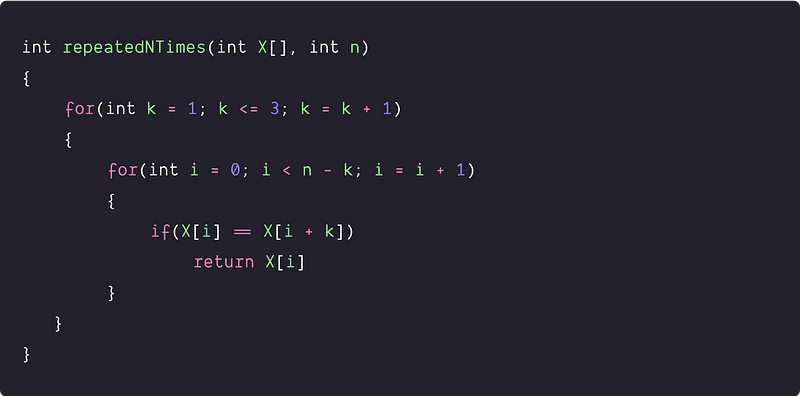# n Repeated element in 2n size array

Key takeaway: An excellent problem to learn optimization using the mathematical approach. Sometimes interesting mathematical insights into the problem can help us to get efficient solutions.

### Let’s understand the problem

In an array X[] of size 2n, there are n+1 unique elements, and exactly one of these elements is repeated n times. Return the element repeated n times.

Examples

Input: X[] = [1, 2, 2, 3], Output: 2

Input: X[] = [2, 1, 2, 5, 3, 2, 2, 4], Output: 2

Input: X[] = [5, 1, 5, 2, 5, 3, 5, 4, 6, 5], Output: 5

### Discussed solution approaches

• A brute force approach  using a hash table
• An efficient approach  using the pigeon hole principle

### A brute force approach using a hash table

Solution Idea

A simple approach would be: Traverse the array and count the frequency of each element using the extra array or using the hash table. During the traversal, if we found the element with a count larger than 1, we return that element. Why are we returning the element with a count larger than 1? (Think!)

Solution PseudocodeTime and space complexity analysis

The traversal of the array takes time proportional to the number of elements in the array. At each iteration, we are doing constant operations because inserting and searching in the hash table takes O(1) time. Hence, Time Complexity = O(n). We are using O(n) extra space to store the frequency of elements in the hash table. Hence, Space Complexity = O(n).

### An efficient approach using the pigeon hole principle

Solution Idea

The idea behind the algorithm is simple: if an element is repeated, then it must be the element that has been repeated n times because all other elements are unique! So, as given in the problem, we know there is an element “x” that appears n times in an array of size 2n. To better understand this algorithm, suppose the indexes of all x’s are the array are a1, a2, ... an, where a1 < a2,…< an. Let us consider the average gap between consecutive x’s.

Average gap = [(a2 - a1) + (a3 - a2) +...(an - a(n-1))] / (n-1). Here a2, a3, ...a(n-1) cancel out each other, so average gap = (an - a1)/(n-1)

As array size is 2n, max possible value of an = 2n - 1, min possible value of a1 = 0. If we put values of an and a1 in the above formula, we can get the maximum upper limit of the average gap i.e. average gap <= (2n - 1)/(n-1).

The value of (2n - 1)/(n-1) <= 3

• Equality holds when n = 2
• for all n > 2, it is less than 3
• So, average gap <= 3

Therefore, from the above analysis, there must exist at least two x’s such that their gap is at most 3. Simply stating: If half the array is repeated, it doesn’t matter how we shuffle it; for one of the repeated elements, there has to be another repeated element at least three positions away; otherwise, the array isn’t 2n.

Solution Steps

• Create three windows of gap lengths 1, 2, and 3.
• For each window, traverse the array and compare the first and last elements of the window.
• If equality holds then, this must be our repeated element.

Solution PseudocodeTime and space complexity analysis

We are using two nested loops where the outer loop is running constant time, i.e., three times only. We are doing one comparison operation at each iteration of the inner loop, i.e., the constant number of operations. So in the worst case, the outer loop runs three times, and we need to traverse each element via the inner loop. Time complexity = Time complexity of the outer loop * Time complexity of the inner loop = O(1)*O(n) = O(n).

We are not using any extra space. Hence, Space complexity = O(1)

### Critical ideas to think!

• What is the pigeonhole principle? How can we use it in problem-solving?
• Can we solve this problem by sorting the input? If yes, then what would be the time and space complexity for this approach?
• Can we solve this problem efficiently using some other approach?
• What would be the worst and best case input for both approaches?
• Can we further optimize the above approach? Is it sufficient to compare each element with its two right neighbors instead of 3?
• Let's design a randomized algorithm — randomly pick two array elements and check whether they come from different cells and have the same value. If they do, then return true. So after how many numbers of repetition algorithm succeeds with probability 1–1/n? Out of n² possible combinations of picking two elements, how many will result in success?

### Comparisons of time and space complexities

• Brute force approach: Time = O(n), Space = O(n)
• Using pigeon hole principle : Time = O(n), Space = O(1)

### Suggested coding problems to practice

• Valid Mountain
• Remove duplicates from sorted array
• A Product Array Puzzle
• Chocolate Distribution Problem
• Best Time to Buy and Sell Stock
• Sort an array in the waveform
• Number of jumps for a thief to cross walls
• Find the number of subarrays with an even sum
• Double the first element and move zero to the end

Thanks to Navtosh Kumar for his contribution in creating the first version of the content. Please write comments if you find an error or you want to share more insights about the topic. Enjoy learning, Enjoy coding, Enjoy algorithms!

Get well-designed application and interview centirc content on ds-algorithms, machine learning, system design and oops. Content will be delivered weekly.